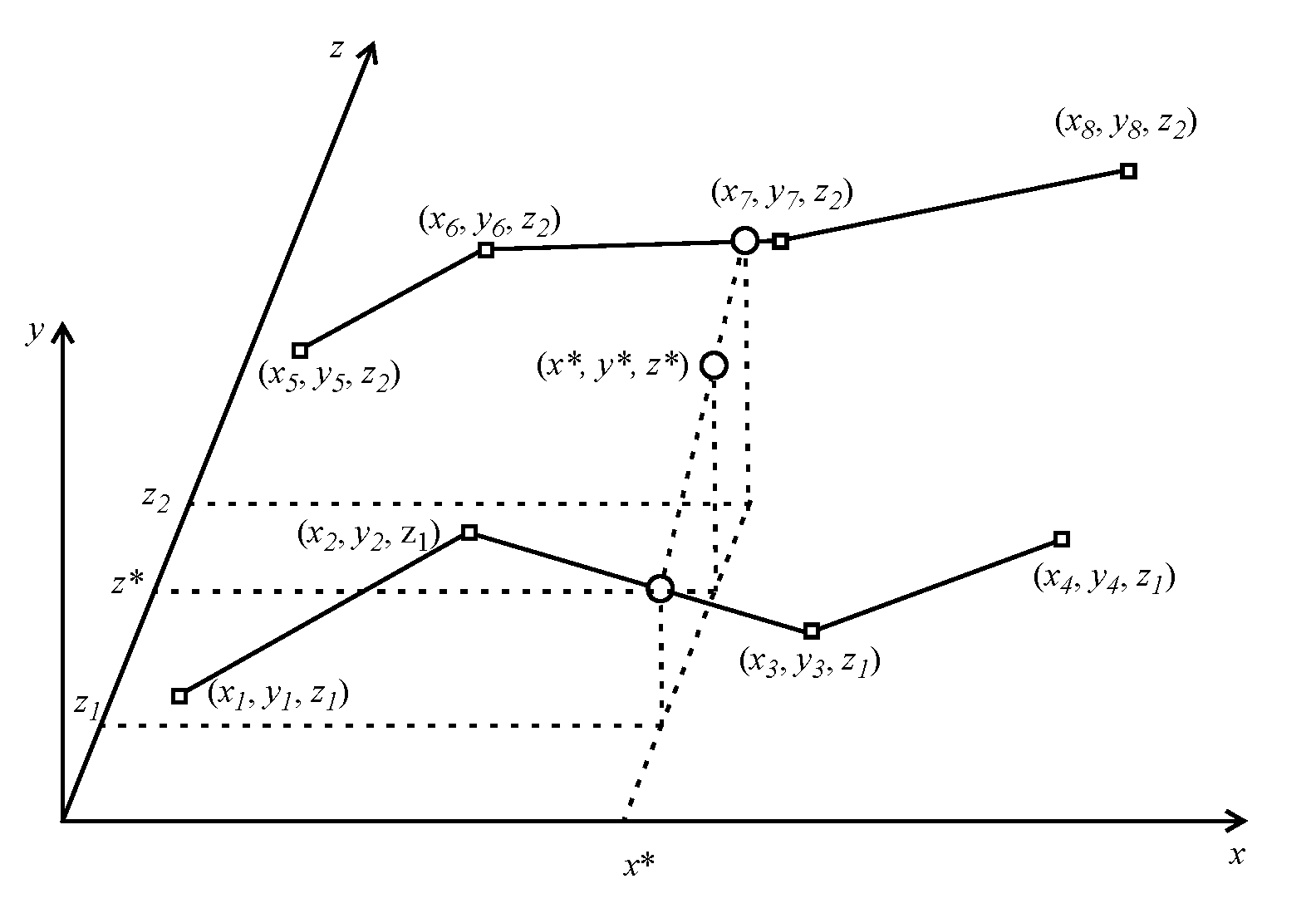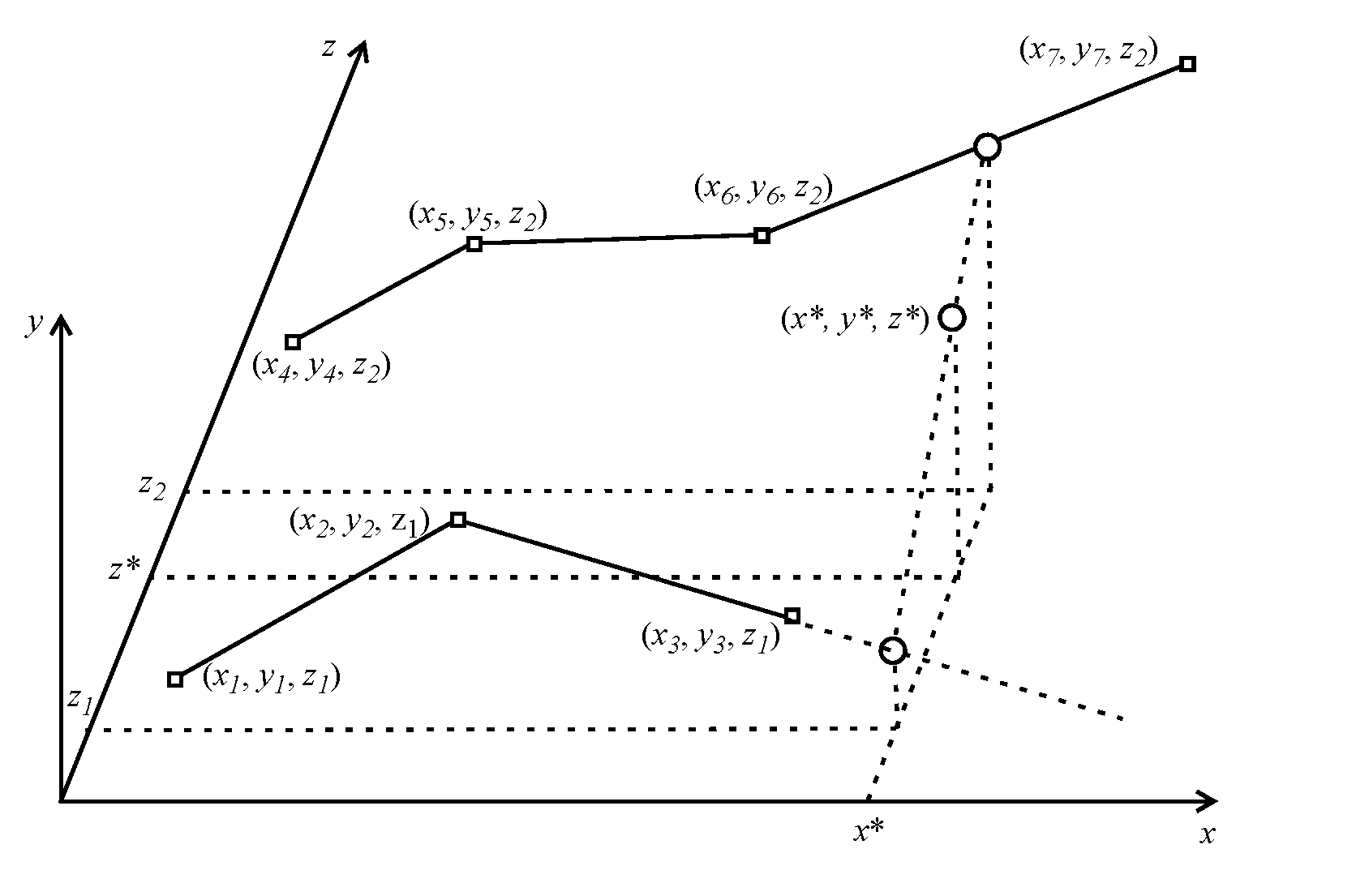Objects and Methods : Table Interpolation : Three-dimensional Table Interpolation
Three-dimensional Table Interpolation
For three-dimensional interpolation, the z values define blocks: each block has a constant z value and increasing x values, and the blocks are arranged in order of increasing z value. In other words, the three-dimensional surface is represented by multiple slices or contours in the x-y plane, each of which may be represented by any arbitrary number of data points, just as with ordinary two-dimensional curves. Table B.2 is an example of the proper way to formulate a table for three-dimensional interpolation.

Table B.2  Plant power table for a power reservoir
Turbine Release (cfs)
Power (kW)
100
0
0
100
10
2000
100
20
3000
100
30
4000
200
0
0
200
10
2500
200
20
3500
200
25
3800
200
30
4500
300
0
0
300
10
3000
300
25
5000
For three-dimensional functions, the algorithm for interpolation has two basic cases, as follows:
• If the z value being interpolated is equal to the z value for one of the blocks in the table, then we just perform a two-dimensional interpolation along the curve represented by that block.
• When the z value is not exactly equal to any of the z values found in the table, RiverWare first identifies the constant z-blocks whose values bound the z value being interpolated and performs a two-dimensional interpolation along these curves. This yields two points, one on each bounding constant z-curve, and the final answer is computed by a linear interpolation between these two points. Figure B.2 illustrates this case. We denote a particular approximation using the table by an asterisk: y* = f(x*, z*)
Figure B.2  Three-dimensional linear interpolationThere is one special case in which the interpolation behavior is slightly different: when the x value being interpolated is within the domain of one of the bracketing constant z curves but not the other. In this case, we interpolate between the encompassing curve and the extrapolation of the other (shorter) curve. We extrapolate this curve with either the slope of its last segment or the slope of the corresponding segment of the encompassing curve, as appropriate for the particular table. To avoid overambitious extrapolation, RiverWare requires that the answer lie in the region bounded by the constant-z curves (that is, their convex hull). Figure B.3 illustrates this case, where the short curve is extrapolated with the slope of its last segment.
Figure B.3  Three-dimensional linear interpolationThree-dimensional Table Interpolation Errors
The following types of errors may be reported during three-dimensional table interpolation:
• Invalid value (data error): an x, y, or z value is invalid (xi = NaN, yi = NaN, or zi = NaN for some i).
• Non-increasing z (data error): the z values are not increasing for one block to another (zi >= zi-1, for some i).
• Non-increasing x (data error): the x values are not increasing (xi >= xi-1, for some i).
• z value out of range (interpolation error): the z value being interpolated is out of the range of the table (z* < zmin or z* > zmax).
• x value out of range (interpolation error): the x value being interpolated is out of the domain of both of the two bounding constant z-curves.

Revised: 08/02/2021